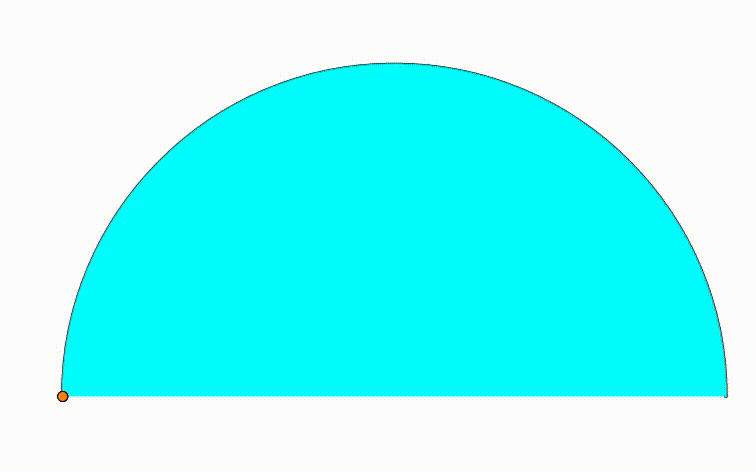# Dynamic Geometry: P85

Geometry Level pendingThe diagram shows a black semicircle. The cyan and green semicircle are growing and shrinking freely on the black semicirle's diameter. The purple circle is internally tangent to the black semicircle and tangent to both green and cyan semicircle. The red and orange circles are internally tangent to the black semicircle and tangent to the purple circle and to one of the bottom semicircles. When the ratio of the red circle's radius to the radius of the orange circle is equal to $\dfrac{123}{43}$, the ratio of the green circle's radius to the radius of the cyan circle can be expressed as $\dfrac{p}{q}$, where $p$ and $q$ are coprime positive integers. Find $p+q$.

×